# Dispersion Trading Using Options [EPAT PROJECT]

This article is the final project submitted by the author as a part of his coursework in Executive Programme in Algorithmic Trading (EPAT™) at QuantInsti®. Do check our Projects page and have a look at what our students are building.

### Strategy

To distinguish dispersion trading, it is simply a hedged strategy which takes advantage of relative value differences in the implied volatilities between an index and index component stocks. It involves a short options position on securities of index and a long option positions on the components of the index or vice versa. Effectively, we will long/short straddles based on our entry signals. We have to note that this trade would be successful only when the delta exposure is close to zero. Thus, the dispersion strategy is hedged against large market movements. Below is the progression of actions to be taken for a successful dispersion trade.

• Calculate the Dirty Correlation (ρ)
• Generate Signals when Dirty Correlation crosses threshold/reaches its extreme
• Buy/Sell Index and individual securities as per the logic
• Compute Delta at regular intervals and offset it by buying/selling futures to make the trade delta neutral
• Exit when Dirty Correlation crosses the mean (ρ=0.5)

To understand how the difference in volatility is captured, we need to understand the variance of index with a basket of Stocks. It is given as: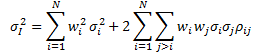where, σI2  is the index variance wi is the weight for stock in the index σi2 is the individual stock variance ρij is the correlation of stock i with stock j The profit from this strategy comes from the fact that correlation tends to mean revert. Thus, if one takes positions during the extremes of ratio, we can be assured that it would mean revert at a certain point.

### Implementing the Strategy

To implement the strategy, we would need to calculate the metrics given below.

#### Calculating Implied volatility of nearest Strikes

Since we have the Premium values, time to expiry, Interest Rate, Dividend and the nearest Strike, we can compute the Implied Volatility of the nearest strikes using the Black Scholes model. The weighted average Implied Volatility among the nearest strikes needs to be added for the individual securities and Index in order to calculate the Correlation.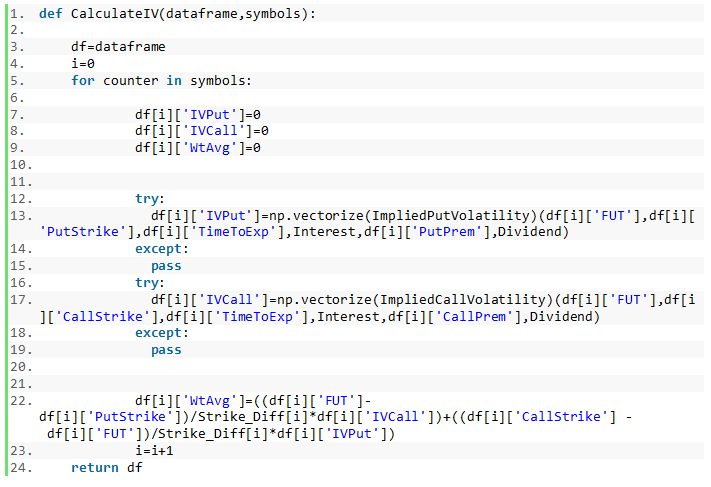In the above function, CalculateIV, we can see that the individual Call and Put IV’s are computed first and then a weighted average of these IV’s are calculated. The weights are given more to those strikes which are nearer to the future price.

#### Dirty Correlation

This is the square of ratio of the Implied Volatility of Index and Weighted average of Stocks. The formula is given as: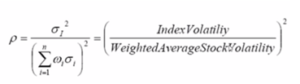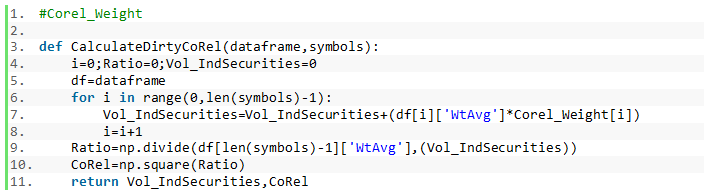The snippet above shows the computation of the dirty correlation. Here the volume of Individual securities is computed first and summed in Vol_IndSecurities. The ratio is computed between index and individual stocks and is squared.

#### Defining Thresholds

This is an important step to generate entry/exit signals based on the risk appetite. In this project, the thresholds are z1=0.2, z2=0.8, z3=0.5 Where, z1 gives the signal to buy Index and short the individual securities z2 gives the signal to short the Index and long individual securities z3 is the exit threshold where all positions are to be squared off

### Choosing Options to be Traded

As soon as we get the signal to buy/sell, we would be using a combination of straddle and strangle of both puts and calls. The nearest 3 OTM strikes are considered in this project. The investment amount needs to be equally split among the Index and Individual securities. While taking an entry, the lot size, the quantity bought needs to be noted down so that the deltas at each stage are handy.

### Hedging

This is further hedged using future contracts to keep the whole process delta neutral. Delta of this strategy should be adjusted every fifteen minutes. When the delta goes above 1, one future contract is sold and when the delta drops to -1 the delta is neutralized by buying one futures contract. It is important to keep the delta close to zero for the duration of the trade.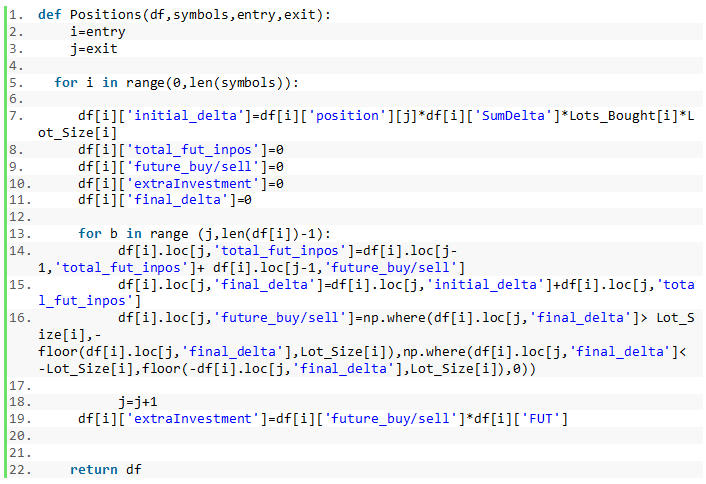The above snippet shows how the positions are taken based on the entry/exit signals. Whenever we have an entry signal for a trade, we constantly monitor the delta to ensure it is neutralized. For the same, the total deltas are summed up initially and they are offset with a futures contract being bought or sold. The futures currently in position are also kept handy to compute the delta for every tick. The total futures bought or sold are identified and multiplied by the futures price to compute the extra investment for the trade.

### Profit and loss Calculation

To calculate the PnL, the following needs to be considered:

• Initial trade, cost at which options were bought/sold when entry was taken
• Hedging cost, the total amount of futures invested to make the trade delta neutral
• Futures Settlement, the amount resulted in settling the futures contract at the exit signal
• Options Square off, the amount resulted when all positions were squared off at exit signal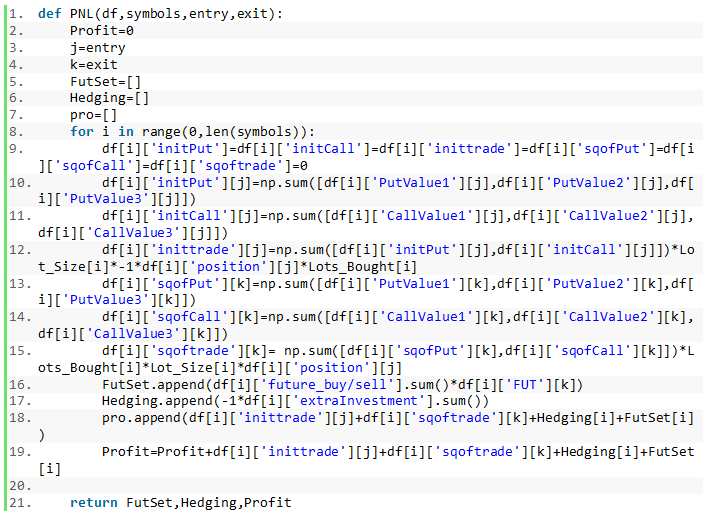The snippet above shows the calculation of PnL. Here we can see that we have computed the cost of purchase of the initial trade (Buying/Selling of Calls and Puts). We have computed the squared off price of the calls and puts. The additional investment or the futures bought or sold is represented in FutSet which is squared off at the end of the trade. The hedging amount is stored in the Hedging variable.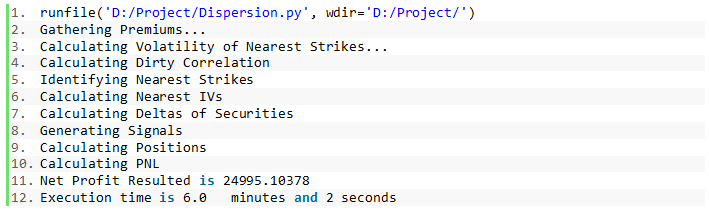Above is the sample execution of the code. As can be seen, the execution takes up considerable time due to complex calculations involved in the script.

### Conclusion

Dispersion trading is a complex strategy, however this is rewarded with the strategy being a profitable one which offers high rewards in response to a low risk. To make this strategy even better, it would be necessary to automate the strategy and the hedging should be dynamic as per the price movements. Trading at times where volatility is high (viz. quarterly results, individual stock news etc.) when the correlation would not be strong may result in more profit. To maximize the accuracy of the strategy, we can decrease the time interval to capture the volatility and accordingly compute deltas. Know about Open Interest In Options Trading an indicator that can easily be used in Futures and Options trading, what open interest indicates, how to read open interest data and considers some basic assumptions about how one can build an Open Interest Trading Using Python